# RD Sharma Solutions for Class 11 Chapter 16 - Permutations Exercise 16.4

In this section, we shall discuss permutations where either repetition of items are allowed or distinction between some of the items are ignored or a particular item occurs in every arrangement etc. such type of permutations are termed as permutations under certain conditions. Subject experts have prepared the solutions to improve confidence among students to appear for the board exam without fear. The solutions pdf are developed based on the requirement of students, which reduces time constraint and helps students solve problems at ease. In order to excel in the board exams with high marks RD Sharma Class 11 Maths Solutions pdf is available here for free download.

## Download the pdf of RD Sharma Solutions for Class 11 Maths Exercise 16.4 Chapter 16 – Permutations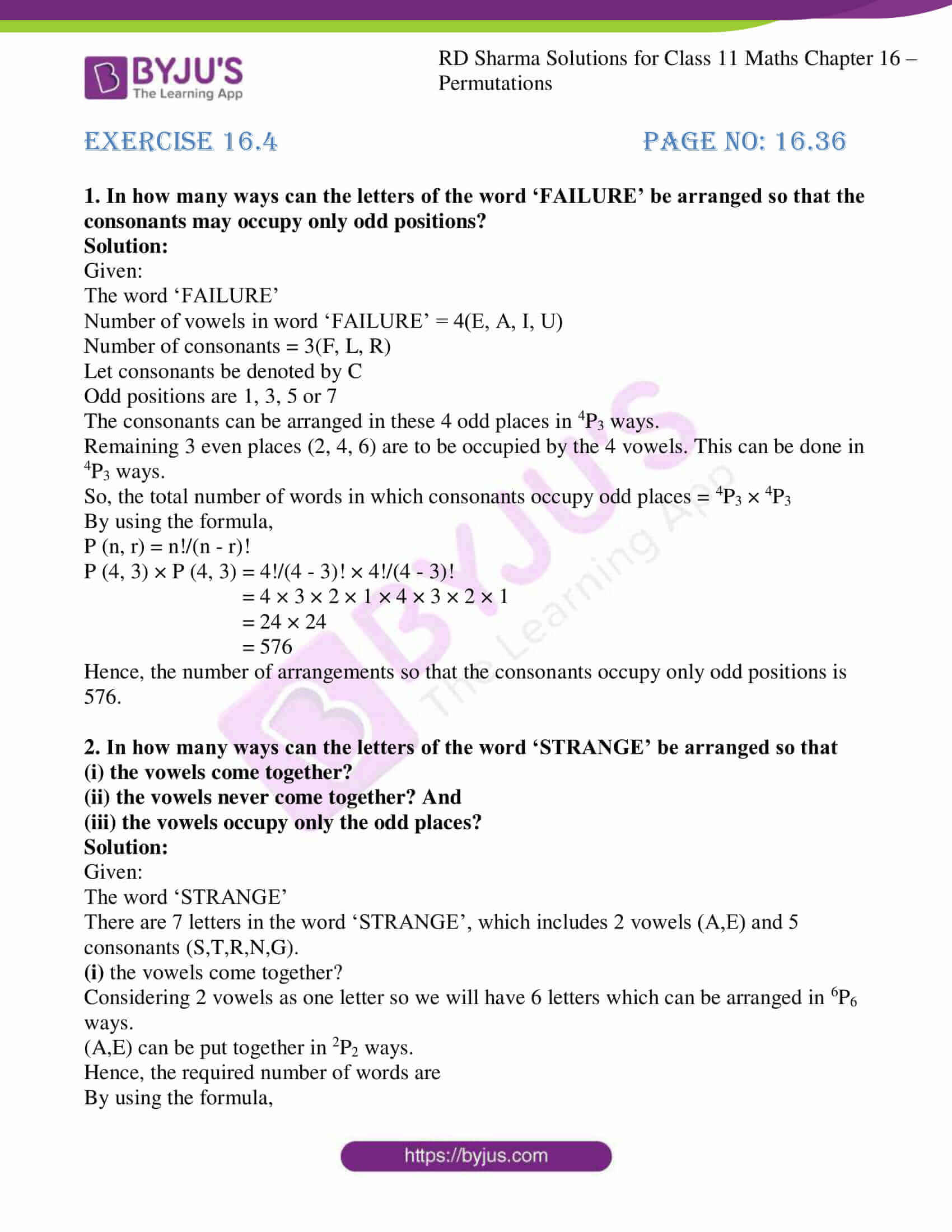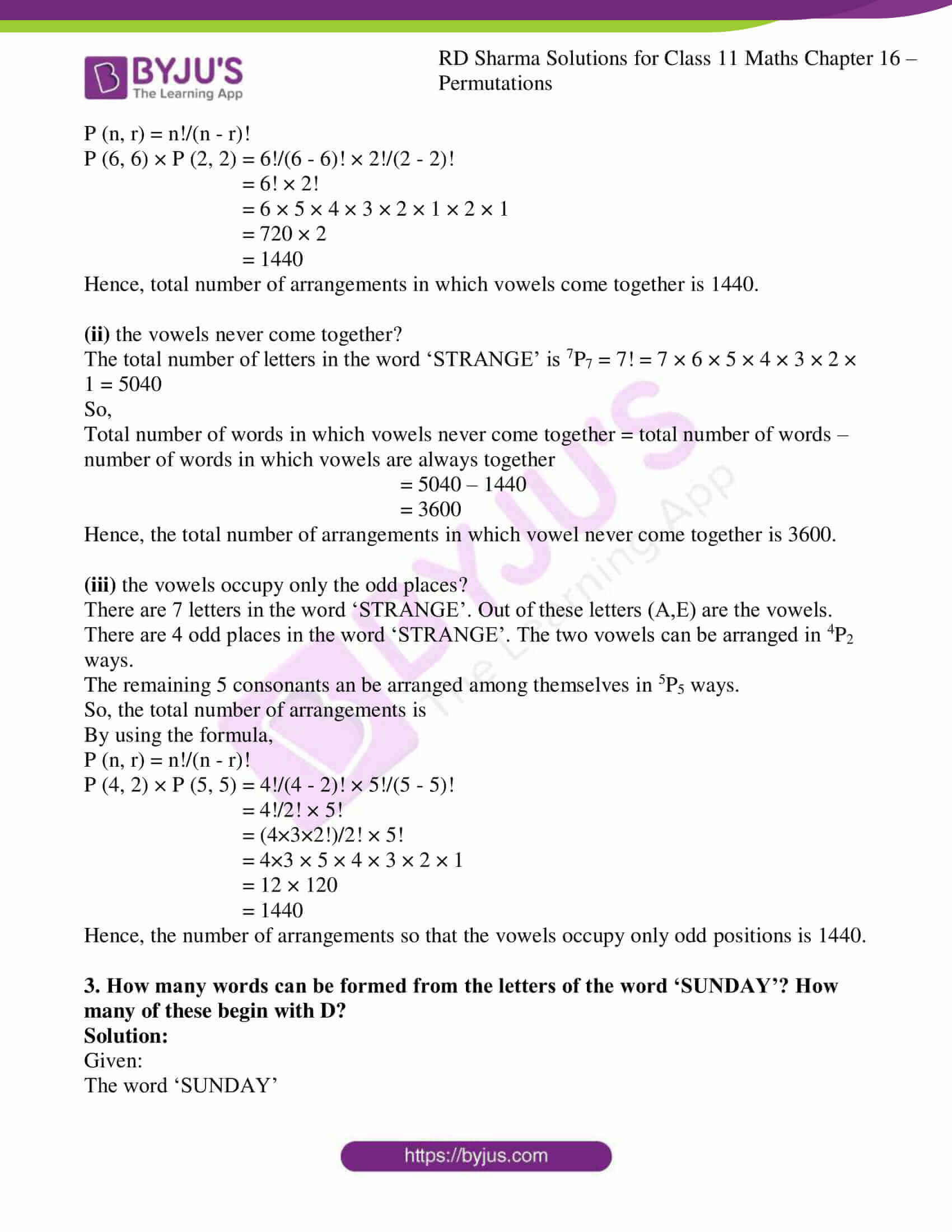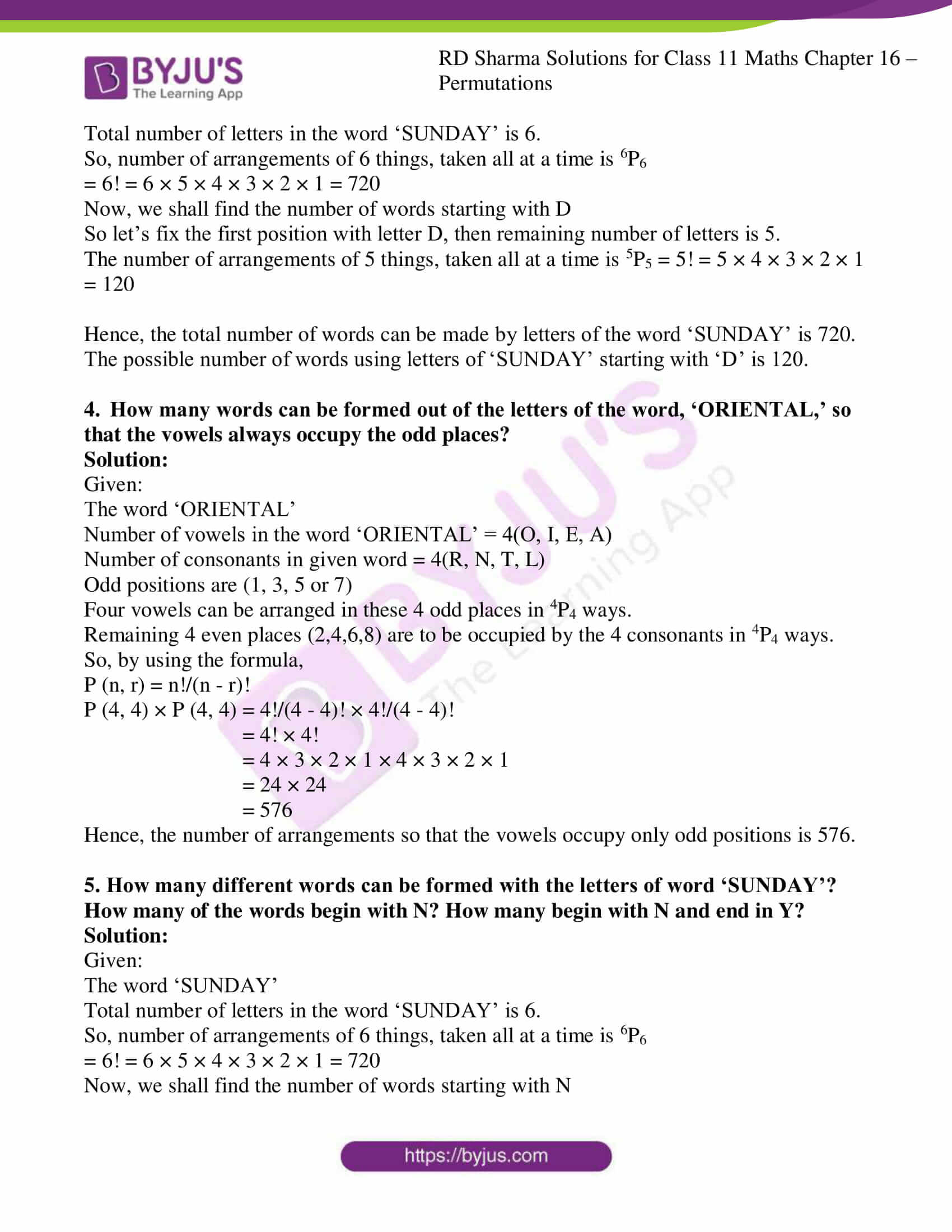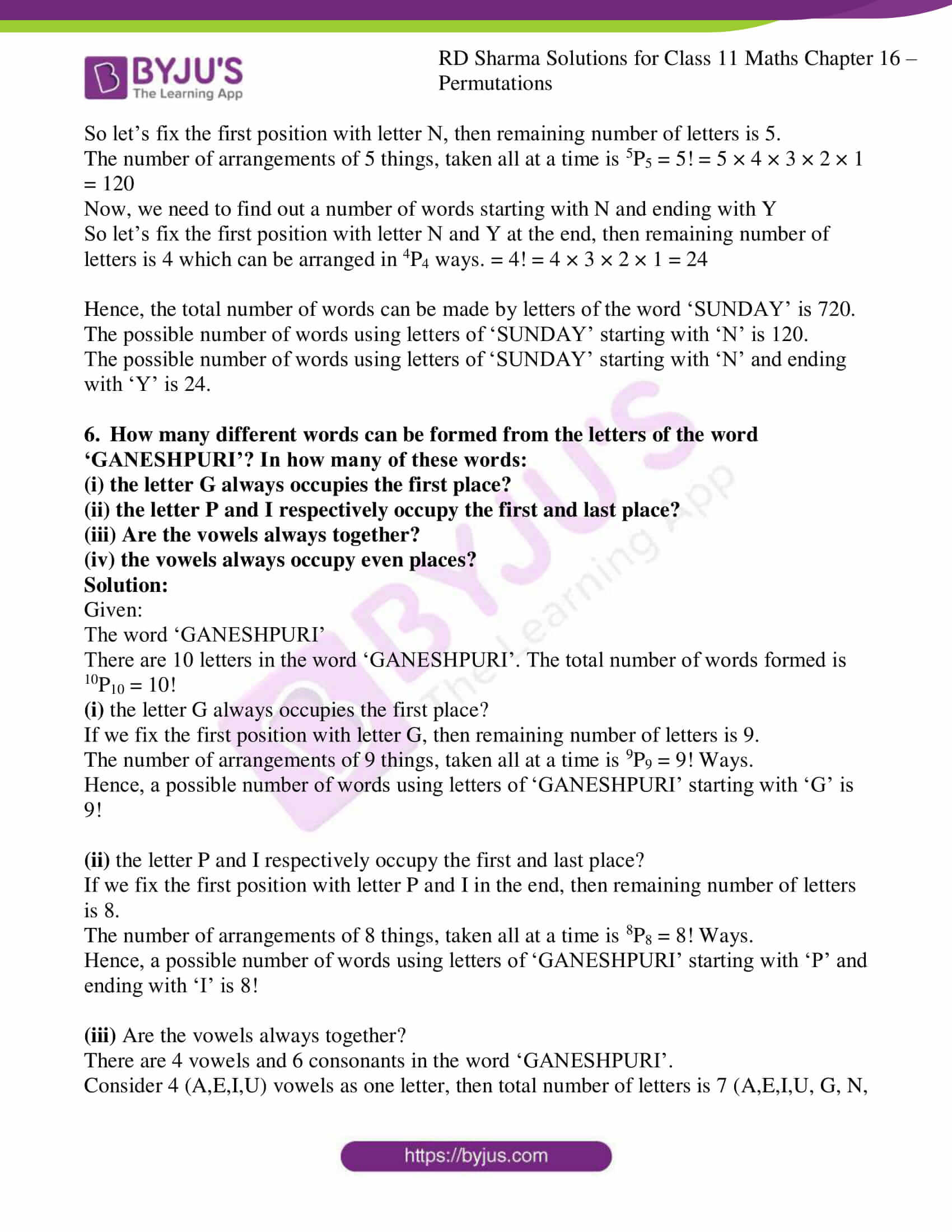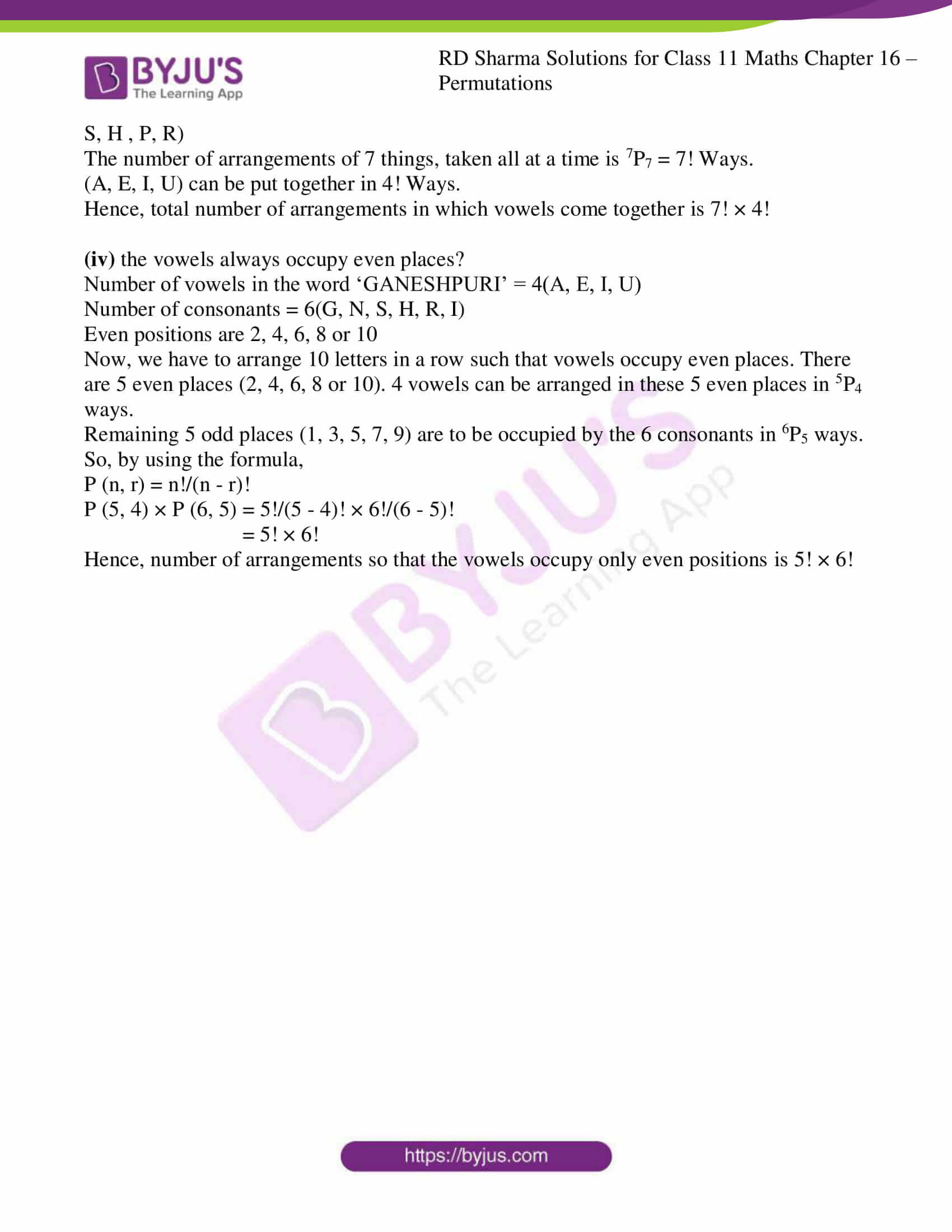### Also, access other exercises of RD Sharma Solutions for Class 11 Maths Chapter 16 – Permutations

Exercise 16.1 Solutions

Exercise 16.2 Solutions

Exercise 16.3 Solutions

Exercise 16.5 Solutions

### Access answers to RD Sharma Solutions for Class 11 Maths Exercise 16.4 Chapter 16 – Permutations

1. In how many ways can the letters of the word ‘FAILURE’ be arranged so that the consonants may occupy only odd positions?

Solution:

Given:

The word ‘FAILURE’

Number of vowels in word ‘FAILURE’ = 4(E, A, I, U)

Number of consonants = 3(F, L, R)

Let consonants be denoted by C

Odd positions are 1, 3, 5 or 7

The consonants can be arranged in these 4 odd places in 4P3 ways.

Remaining 3 even places (2, 4, 6) are to be occupied by the 4 vowels. This can be done in 4P3 ways.

So, the total number of words in which consonants occupy odd places = 4P3 × 4P3

By using the formula,

P (n, r) = n!/(n – r)!

P (4, 3) × P (4, 3) = 4!/(4 – 3)! × 4!/(4 – 3)!

= 4 × 3 × 2 × 1 × 4 × 3 × 2 × 1

= 24 × 24

= 576

Hence, the number of arrangements so that the consonants occupy only odd positions is 576.

2. In how many ways can the letters of the word ‘STRANGE’ be arranged so that
(i) the vowels come together?

(ii) the vowels never come together? And

(iii) the vowels occupy only the odd places?

Solution:

Given:

The word ‘STRANGE’

There are 7 letters in the word ‘STRANGE’, which includes 2 vowels (A,E) and 5 consonants (S,T,R,N,G).

(i) the vowels come together?

Considering 2 vowels as one letter so we will have 6 letters which can be arranged in 6P6 ways.

(A,E) can be put together in 2P2 ways.

Hence, the required number of words are

By using the formula,

P (n, r) = n!/(n – r)!

P (6, 6) × P (2, 2) = 6!/(6 – 6)! × 2!/(2 – 2)!

= 6! × 2!

= 6 × 5 × 4 × 3 × 2 × 1 × 2 × 1

= 720 × 2

= 1440

Hence, total number of arrangements in which vowels come together is 1440.

(ii) the vowels never come together?

The total number of letters in the word ‘STRANGE’ is 7P7 = 7! = 7 × 6 × 5 × 4 × 3 × 2 × 1 = 5040

So,

Total number of words in which vowels never come together = total number of words – number of words in which vowels are always together

= 5040 – 1440

= 3600

Hence, the total number of arrangements in which vowel never come together is 3600.

(iii) the vowels occupy only the odd places?

There are 7 letters in the word ‘STRANGE’. Out of these letters (A,E) are the vowels.

There are 4 odd places in the word ‘STRANGE’. The two vowels can be arranged in 4P2 ways.

The remaining 5 consonants an be arranged among themselves in 5P5 ways.

So, the total number of arrangements is

By using the formula,

P (n, r) = n!/(n – r)!

P (4, 2) × P (5, 5) = 4!/(4 – 2)! × 5!/(5 – 5)!

= 4!/2! × 5!

= (4×3×2!)/2! × 5!

= 4×3 × 5 × 4 × 3 × 2 × 1

= 12 × 120

= 1440

Hence, the number of arrangements so that the vowels occupy only odd positions is 1440.

3. How many words can be formed from the letters of the word ‘SUNDAY’? How many of these begin with D?

Solution:

Given:

The word ‘SUNDAY’

Total number of letters in the word ‘SUNDAY’ is 6.

So, number of arrangements of 6 things, taken all at a time is 6P6

= 6! = 6 × 5 × 4 × 3 × 2 × 1 = 720

Now, we shall find the number of words starting with D

So let’s fix the first position with letter D, then remaining number of letters is 5.

The number of arrangements of 5 things, taken all at a time is 5P5 = 5! = 5 × 4 × 3 × 2 × 1 = 120

Hence, the total number of words can be made by letters of the word ‘SUNDAY’ is 720.

The possible number of words using letters of ‘SUNDAY’ starting with ‘D’ is 120.

4. How many words can be formed out of the letters of the word, ‘ORIENTAL,’ so that the vowels always occupy the odd places?

Solution:

Given:

The word ‘ORIENTAL’

Number of vowels in the word ‘ORIENTAL’ = 4(O, I, E, A)

Number of consonants in given word = 4(R, N, T, L)

Odd positions are (1, 3, 5 or 7)

Four vowels can be arranged in these 4 odd places in 4P4 ways.

Remaining 4 even places (2,4,6,8) are to be occupied by the 4 consonants in 4P4 ways.

So, by using the formula,

P (n, r) = n!/(n – r)!

P (4, 4) × P (4, 4) = 4!/(4 – 4)! × 4!/(4 – 4)!

= 4! × 4!

= 4 × 3 × 2 × 1 × 4 × 3 × 2 × 1

= 24 × 24

= 576

Hence, the number of arrangements so that the vowels occupy only odd positions is 576.

5. How many different words can be formed with the letters of word ‘SUNDAY’? How many of the words begin with N? How many begin with N and end in Y?

Solution:

Given:

The word ‘SUNDAY’

Total number of letters in the word ‘SUNDAY’ is 6.

So, number of arrangements of 6 things, taken all at a time is 6P6

= 6! = 6 × 5 × 4 × 3 × 2 × 1 = 720

Now, we shall find the number of words starting with N

So let’s fix the first position with letter N, then remaining number of letters is 5.

The number of arrangements of 5 things, taken all at a time is 5P5 = 5! = 5 × 4 × 3 × 2 × 1 = 120

Now, we need to find out a number of words starting with N and ending with Y

So let’s fix the first position with letter N and Y at the end, then remaining number of letters is 4 which can be arranged in 4P4 ways. = 4! = 4 × 3 × 2 × 1 = 24

Hence, the total number of words can be made by letters of the word ‘SUNDAY’ is 720.

The possible number of words using letters of ‘SUNDAY’ starting with ‘N’ is 120.

The possible number of words using letters of ‘SUNDAY’ starting with ‘N’ and ending with ‘Y’ is 24.

6. How many different words can be formed from the letters of the word ‘GANESHPURI’? In how many of these words:
(i) the letter G always occupies the first place?

(ii) the letter P and I respectively occupy the first and last place?

(iii) Are the vowels always together?

(iv) the vowels always occupy even places?

Solution:

Given:

The word ‘GANESHPURI’

There are 10 letters in the word ‘GANESHPURI’. The total number of words formed is 10P10 = 10!

(i) the letter G always occupies the first place?

If we fix the first position with letter G, then remaining number of letters is 9.

The number of arrangements of 9 things, taken all at a time is 9P9 = 9! Ways.

Hence, a possible number of words using letters of ‘GANESHPURI’ starting with ‘G’ is 9!

(ii) the letter P and I respectively occupy the first and last place?

If we fix the first position with letter P and I in the end, then remaining number of letters is 8.

The number of arrangements of 8 things, taken all at a time is 8P8 = 8! Ways.

Hence, a possible number of words using letters of ‘GANESHPURI’ starting with ‘P’ and ending with ‘I’ is 8!

(iii) Are the vowels always together?

There are 4 vowels and 6 consonants in the word ‘GANESHPURI’.

Consider 4 (A,E,I,U) vowels as one letter, then total number of letters is 7 (A,E,I,U, G, N, S, H , P, R)

The number of arrangements of 7 things, taken all at a time is 7P7 = 7! Ways.

(A, E, I, U) can be put together in 4! Ways.

Hence, total number of arrangements in which vowels come together is 7! × 4!

(iv) the vowels always occupy even places?

Number of vowels in the word ‘GANESHPURI’ = 4(A, E, I, U)

Number of consonants = 6(G, N, S, H, R, I)

Even positions are 2, 4, 6, 8 or 10

Now, we have to arrange 10 letters in a row such that vowels occupy even places. There are 5 even places (2, 4, 6, 8 or 10). 4 vowels can be arranged in these 5 even places in 5P4 ways.

Remaining 5 odd places (1, 3, 5, 7, 9) are to be occupied by the 6 consonants in 6P5 ways.

So, by using the formula,

P (n, r) = n!/(n – r)!

P (5, 4) × P (6, 5) = 5!/(5 – 4)! × 6!/(6 – 5)!

= 5! × 6!

Hence, number of arrangements so that the vowels occupy only even positions is 5! × 6!